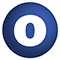# SparkSchema

### Related Doc: package schema

#### object SparkSchema

Schema modification and information retrieval methods.

Linear Supertypes
AnyRef, Any
Ordering
1. Alphabetic
2. By Inheritance
Inherited
1. SparkSchema
2. AnyRef
3. Any
1. Hide All
2. Show All
Visibility
1. Public
2. All

### Value Members

1. #### final def !=(arg0: Any): Boolean

Definition Classes
AnyRef → Any
2. #### final def ##(): Int

Definition Classes
AnyRef → Any
3. #### final def ==(arg0: Any): Boolean

Definition Classes
AnyRef → Any
4. #### final def asInstanceOf[T0]: T0

Definition Classes
Any
5. #### def clone(): AnyRef

Attributes
protected[java.lang]
Definition Classes
AnyRef
Annotations
@throws( ... )
6. #### final def eq(arg0: AnyRef): Boolean

Definition Classes
AnyRef
7. #### def equals(arg0: Any): Boolean

Definition Classes
AnyRef → Any
8. #### def finalize(): Unit

Attributes
protected[java.lang]
Definition Classes
AnyRef
Annotations
@throws( classOf[java.lang.Throwable] )
9. #### final def getClass(): Class[_]

Definition Classes
AnyRef → Any
10. #### def getLabelColumnName: (StructType, String) ⇒ String

Gets the label column name.

Gets the label column name.

returns

The label column name.

11. #### def getLabelColumnName(dataset: DataFrame, modelName: String): String

Gets the label column name.

Gets the label column name.

dataset

The dataset to get the label column from.

modelName

The model to retrieve the label column from.

returns

The label column name.

12. #### def getScoreValueKind(schema: StructType, modelName: String, columnName: String): String

Gets the score value kind or null if it does not exist from the schema.

Gets the score value kind or null if it does not exist from the schema.

schema

The schema to get the score column kind column name from.

modelName

The model to retrieve the score column kind column name from.

columnName

The column to retrieve the score value kind from.

13. #### def getScoreValueKind(dataset: DataFrame, modelName: String, columnName: String): String

Gets the score value kind or null if it does not exist from a dataset.

Gets the score value kind or null if it does not exist from a dataset.

dataset

The dataset to get the score column kind column name from.

modelName

The model to retrieve the score column kind column name from.

columnName

The column to retrieve the score value kind from.

14. #### def getScoredLabelsColumnName: (StructType, String) ⇒ String

Gets the scored labels column name.

Gets the scored labels column name.

returns

The scored labels column name.

15. #### def getScoredLabelsColumnName(dataset: DataFrame, modelName: String): String

Gets the scored labels column name.

Gets the scored labels column name.

dataset

The dataset to get the scored labels column from.

modelName

The model to retrieve the scored labels column from.

returns

The scored labels column name.

16. #### def getScoredProbabilitiesColumnName: (StructType, String) ⇒ String

Gets the scored probabilities column name.

Gets the scored probabilities column name.

returns

The scored probabilities column name.

17. #### def getScoredProbabilitiesColumnName(dataset: DataFrame, modelName: String): String

Gets the scored probabilities column name.

Gets the scored probabilities column name.

dataset

The dataset to get the scored probabilities column from.

modelName

The model to retrieve the scored probabilities column from.

returns

The scored probabilities column name.

18. #### def getScoresColumnName: (StructType, String) ⇒ String

Gets the scores column name.

Gets the scores column name.

returns

The scores column name.

19. #### def getScoresColumnName(dataset: DataFrame, modelName: String): String

Gets the scores column name.

Gets the scores column name.

dataset

The dataset to get the scores column from.

modelName

The model to retrieve the scores column from.

returns

The scores column name.

20. #### def hashCode(): Int

Definition Classes
AnyRef → Any
21. #### def isBoolean(df: DataFrame, column: String): Boolean

Find if the given column is boolean

22. #### def isCategorical(df: DataFrame, column: String): Boolean

Find if the given column is Categorical; use CategoricalColumnInfo for more details

23. #### final def isInstanceOf[T0]: Boolean

Definition Classes
Any
24. #### def isNumeric(df: DataFrame, column: String): Boolean

Find if the given column is numeric

25. #### def isString(df: DataFrame, column: String): Boolean

Find if the given column is a string

26. #### final def ne(arg0: AnyRef): Boolean

Definition Classes
AnyRef
27. #### final def notify(): Unit

Definition Classes
AnyRef
28. #### final def notifyAll(): Unit

Definition Classes
AnyRef
29. #### def setLabelColumnName: (DataFrame, String, String, String) ⇒ DataFrame

Sets the label column name.

Sets the label column name.

returns

The modified dataset.

30. #### def setScoredLabelsColumnName: (DataFrame, String, String, String) ⇒ DataFrame

Sets the scored labels column name.

Sets the scored labels column name.

returns

The modified dataset.

31. #### def setScoredProbabilitiesColumnName: (DataFrame, String, String, String) ⇒ DataFrame

Sets the scored probabilities column name.

Sets the scored probabilities column name.

returns

The modified dataset.

32. #### def setScoresColumnName: (DataFrame, String, String, String) ⇒ DataFrame

Sets the scores column name.

Sets the scores column name.

returns

The modified dataset.

33. #### final def synchronized[T0](arg0: ⇒ T0): T0

Definition Classes
AnyRef
34. #### def toString(): String

Definition Classes
AnyRef → Any
35. #### final def wait(): Unit

Definition Classes
AnyRef
Annotations
@throws( ... )
36. #### final def wait(arg0: Long, arg1: Int): Unit

Definition Classes
AnyRef
Annotations
@throws( ... )
37. #### final def wait(arg0: Long): Unit

Definition Classes
AnyRef
Annotations
@throws( ... )